<< Summary

# Read analog data, in Java, part III## Analog to Digital Converter: Monitor a 12 Volt system

We have seen in the previous documents (available here) how to read and monitor a varying power source from the Raspberry PI using a chip MCP3008.
The power source was in those cases provided by the Raspberry PI itself. Those voltages range from 1.2 Volt (at the CPU level), to 5 Volts (power supply, pin #2 on the GPIO Header), the GPIO pins deliver a 3.3 Volts output.
The MCP3008 itself would not take any current greater than 5 Volts.
If we want to monitor a 12 Volt system (on a boat, an RV, a solar panel...), we will need to use some voltage divider, in order not to overload - and fry - the components we will use.
This divider will sit between the power source to monitor, and the pins CH0 to CH7 of the MCP3008.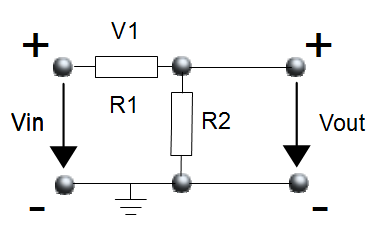The divider

In the figure above:
• `Vin` is the 12 Volt system to monitor. It can go up to 15 Volts.
• `Vout` is what will be used from the MCP3008 standpoint. The + terminal will go to its `CH[0-7]` pins.
`V1` is the tension across the resistor `R1`.
`Vout` (which can also be named `V2`) is the tension across the resistor `R2`.

We (should) know that
 V1 = Vin × R1 and V2 = Vin × R2 R1 + R2 R1 + R2
If we go with:
• Vin = 15 V
• R1 = 100 kΩ
• R2 = 33 kΩ
we find out that Vout = 3.72 V, which is suitable for the MCP3008. We will go with those values.
 Vin V R1 kΩ R2 kΩ Vout 3.72 V

## The setup

Here is the full setup. Notice the following elements:

• The wiring on the Raspberry PI, very close to what it was before, but with some differences regarding the hot wires
• The potentiometer and the voltmeter, which will be used for calibration
• The ground, common to the Raspberry PI and the divider.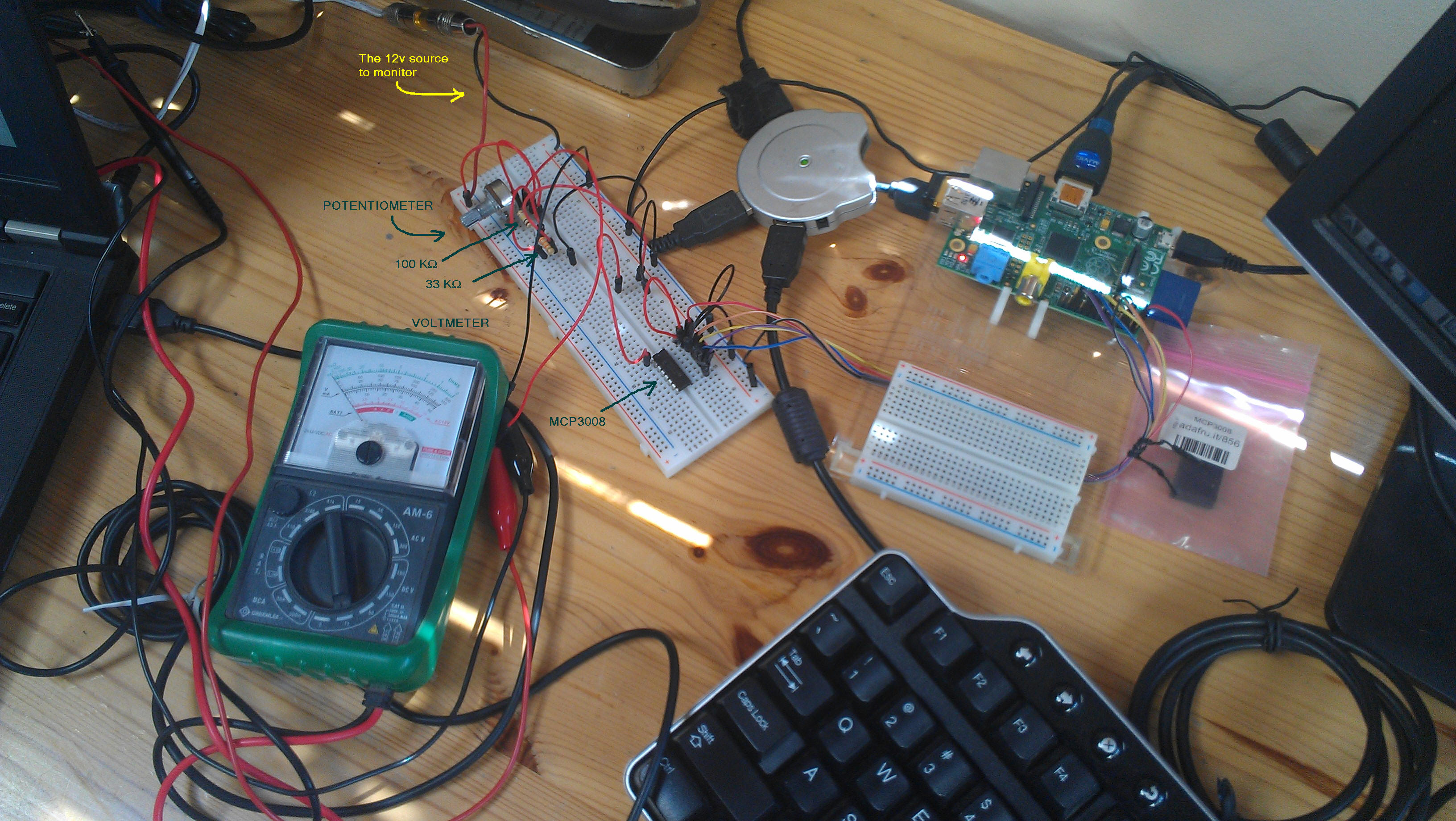All set with the hardware.

## Calibration

A Note
This calibration step is not mandatory. Its goal is to display voltage values as close as possible to the reality. If you are only interested in seeing the way the voltage varies, you can skip it.

The MCP3008 returns values ranging from 0 to 1023 (210 = 1024).
We need to calibrate the hardware so we can directly read the voltage from the program running on the Raspberry PI.
To do so, with the setup above, on the Raspberry PI, start the following script:

```
Prompt> ./battery.monitor -calibration
```
Turn the potentiometer all the way counter-clockwise
```
Prompt> ./battery.monitor -calibration
Parameters are:
-calibration or -cal
-debug=y|n|yes|no|true|false - example -debug=y        (default is n)
-ch=[0-7]                    - example -ch=0           (default is 0)
-min=minADC:minVolt          - example -min=280:3.75   (default is    0:0.0)
-max=maxADC:maxVolt          - example -min=879:11.25  (default is 1023:15.0)
-tune=ADC:volt               - example -tune=973:12.6  (default is 1023:15.0)
-scale=y|n                   - example -scale=y        (default is n)
-log=[log-file-name]         - example -log=[batt.csv] (default is battery.log)
```
Read the voltmeter: In this example, let's say that it displays 3.75 Volts, when the ADC value is at 289.
Now, turn the potentiometer all the way clockwise, and read the voltmeter:
```
...
```
In this example, the voltmeter reads 11.75 Volts when the ADC says 872.
To summarize:
 ADC Voltmeter Mini 289 3.75 Maxi 872 11.75
Now, re-run the script as below. Notice the `-min` and `-max` parameters, and how they relate to the table above. Move the potentiometer back and forth.
```
Prompt> ./battery.monitor -min=289:3.75 -max=872:11.75 -debug=y
Parameters are:
-calibration or -cal
-debug=y|n|yes|no|true|false - example -debug=y        (default is n)
-ch=[0-7]                    - example -ch=0           (default is 0)
-min=minADC:minVolt          - example -min=280:3.75   (default is    0:0.0)
-max=maxADC:maxVolt          - example -min=879:11.25  (default is 1023:15.0)
-tune=ADC:volt               - example -tune=973:12.6  (default is 1023:15.0)
-scale=y|n                   - example -scale=y        (default is n)
-log=[log-file-name]         - example -log=[batt.csv] (default is battery.log)
```
The voltage displayed on the screen should match what's read on the voltmeter.

## Two calibration options

You can use 2 points as above, or one only.
In the case you use only one, we will assume that for 0 ADC, we have 0 Volts.
Let us see.
If we use the following calibration parameters:

```
-min=695:9.1 -max=973:12.6 -scale=y
```
The voltage ranges from `-0.3500028 V` for ADC=0, to `13.265468 V` for ADC=1023. This produces the following figure.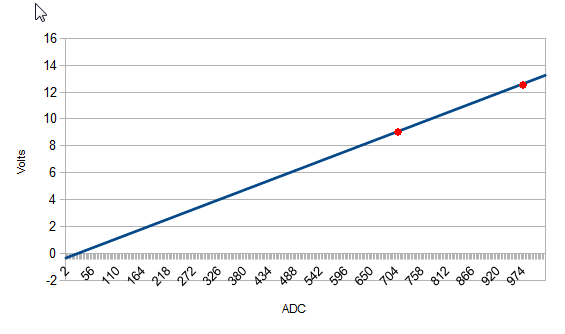If we use:
```
-tune=973:12.6 -scale=y
```
The voltage ranges from `0.0 V` to `13.247482 V` for ADC=1023, and produces the following figure.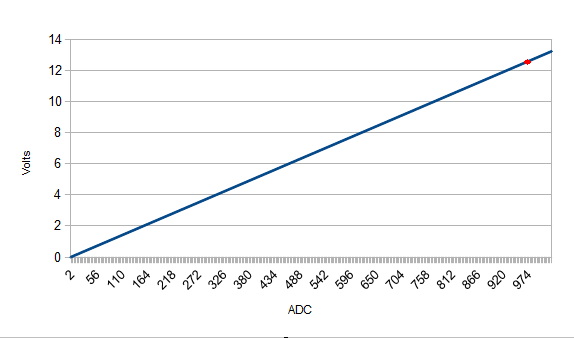This seems good enough.

## Operation

Run the script without the `-debug` parameter.

```
Prompt>./battery.monitor -tune=973:12.6
Parameters are:
-calibration or -cal
-debug=y|n|yes|no|true|false - example -debug=y        (default is n)
-ch=[0-7]                    - example -ch=0           (default is 0)
-min=minADC:minVolt          - example -min=280:3.75   (default is    0:0.0)
-max=maxADC:maxVolt          - example -min=879:11.25  (default is 1023:15.0)
-tune=ADC:volt               - example -tune=973:12.6  (default is 1023:15.0)
-scale=y|n                   - example -scale=y        (default is n)
-log=[log-file-name]         - example -log=[batt.csv] (default is battery.log)

....

^C
Shutting down
Closing log file
Shutting down...
Prompt>
```
Let it run as long as you want, and stop it with a SIGTERM (Ctrl+C).
The data are logged in the file named `battery.log`, as CSV data. Columns are `date`, `ADC`, `Volts`.
```
Prompt> tail battery.log
2014-04-19 11:45:39.804;523;6.9609776
2014-04-19 11:45:39.907;558;7.441252
2014-04-19 11:45:40.009;589;7.866638
2014-04-19 11:45:40.112;636;8.511578
2014-04-19 11:45:40.229;681;9.129074
2014-04-19 11:45:40.331;736;9.883791
2014-04-19 11:45:40.439;788;10.597342
2014-04-19 11:45:40.542;850;11.448113
2014-04-19 11:45:40.645;875;11.791166
2014-04-19 11:45:41.455;869;11.708834
Prompt>
```

## Slice of Pi

Once the prototyping phase is validated, all the components are set on a Slice of PI.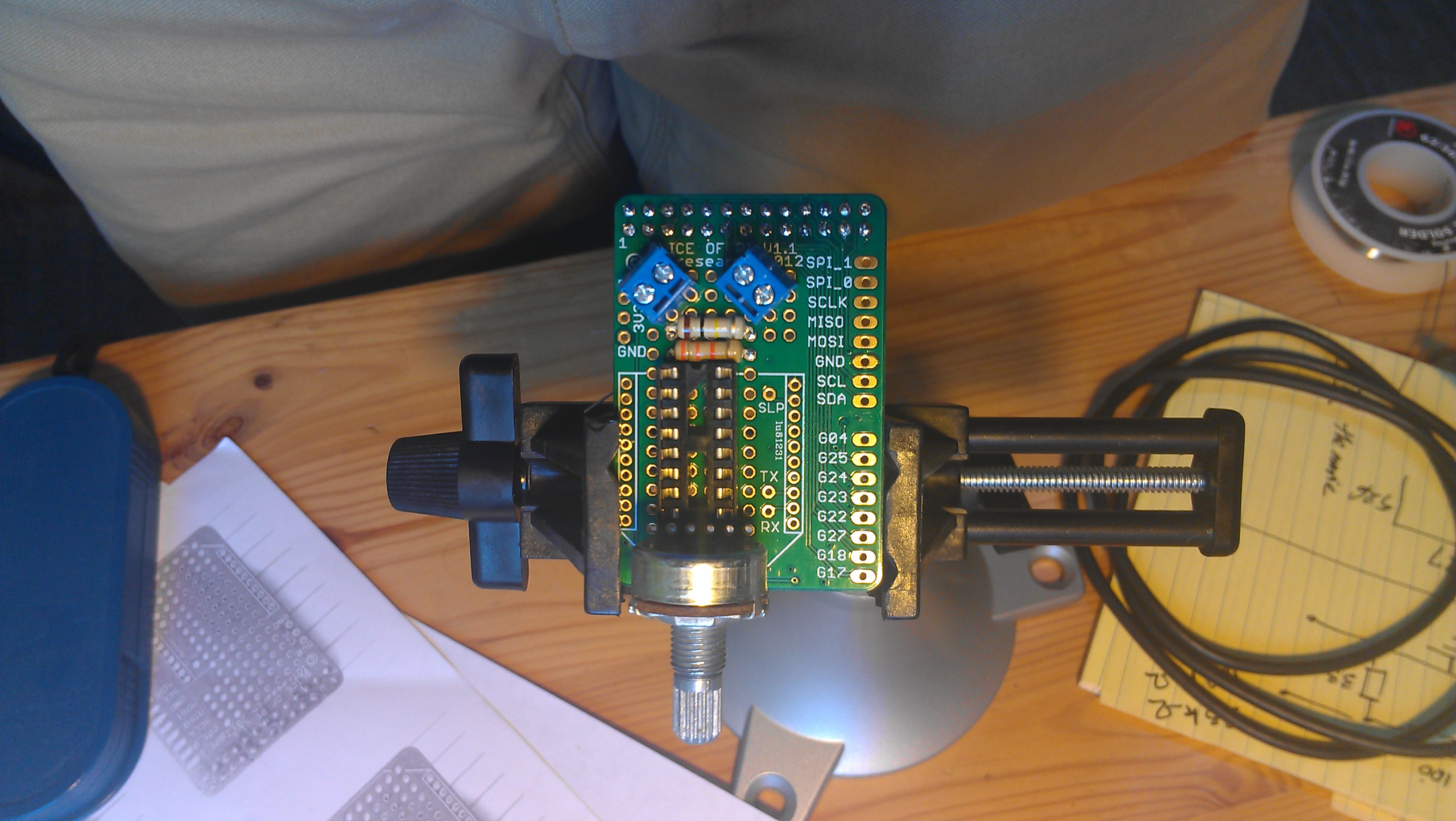The components on the board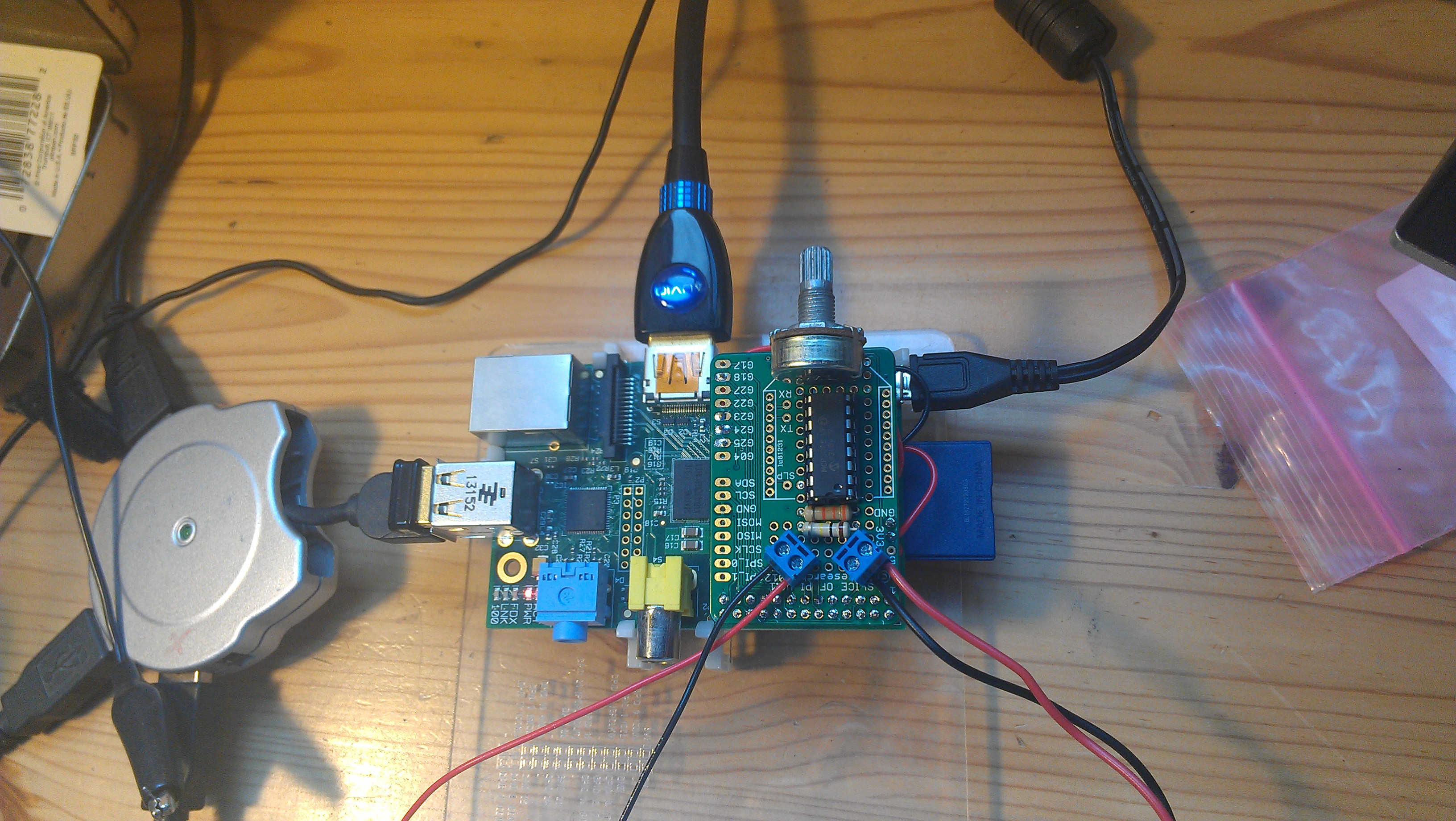Wired. At work.

One terminal is used to hook up the 12v system to monitor, the other is used for calibration, with the voltmeter.

## Logging and rendering

The logging is performed as above.

The data in red are raw data, the blue curve is the result of a smoothing process.
The big spike downward is the starter of the engine...

## Version 2

After a couple of tests, there was some room for improvement, on the software side mostly.
On the hardware side though, a smaller potentiometer considerably reduced the height of the device, and it fits in the chart table.
Also, it could be a good idea to leave some possibility to use some I2C board. As the Slice of PI uses the full GPIO header, four wires have been soldered (GND, 3V3, SCL, SDA), they could be plugged onto a breadboard to use breakout boards like a BMP180, for example.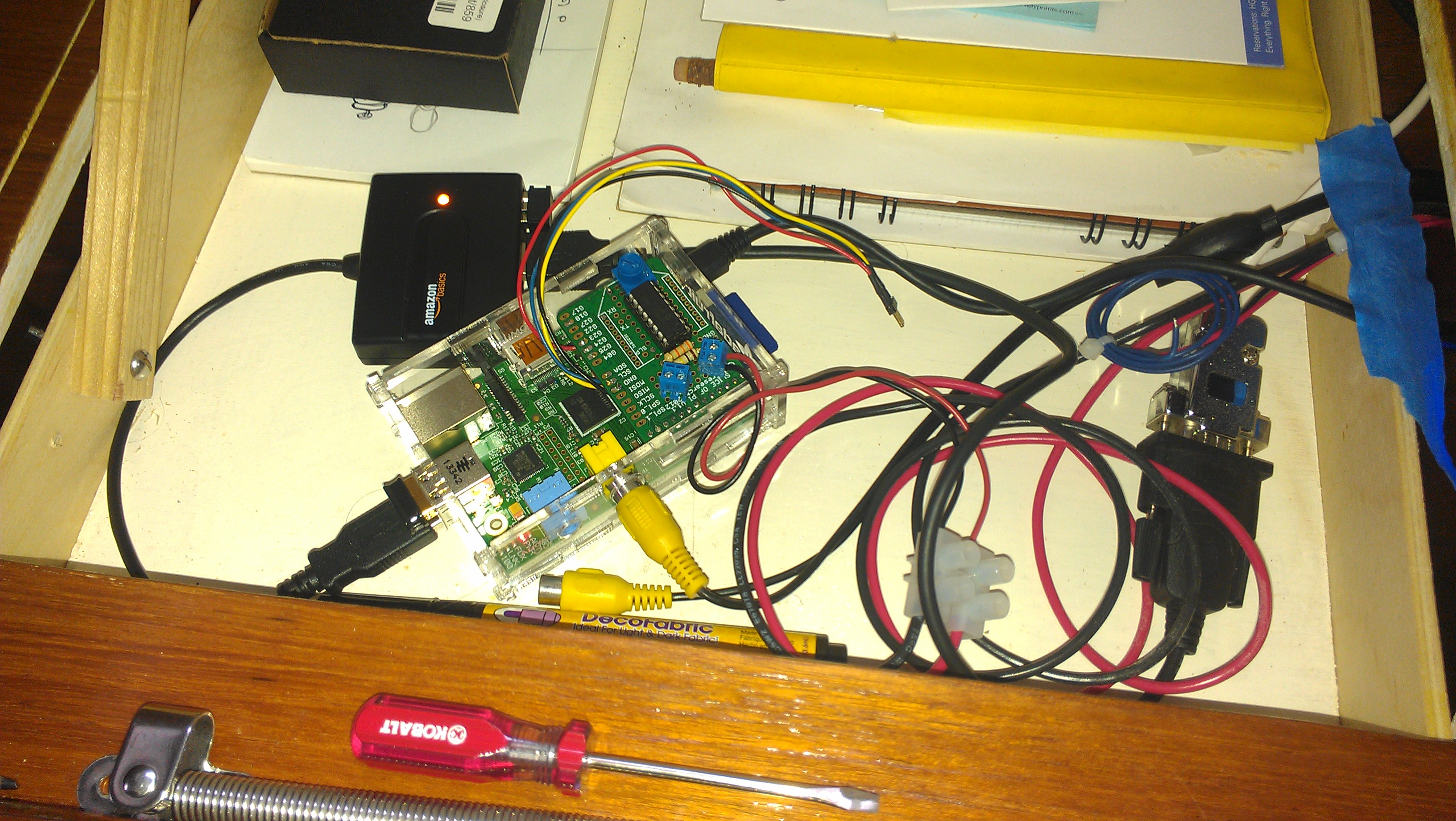In the chart table. Smaller potentiometer, an I2C extension. NMEA Logging.

And NMEA multiplexing can be used as well. There is - as far as I know - no NMEA String for the battery tension..., I just came up with my own. From there, regular NMEA logging can be used:
```
\$IIMTW,+14.5,C*38
\$IIMWV,151,R,04.4,N,A*16
\$IIMWV,150,T,04.5,N,A*10
\$IIRMC,030143,A,3730.078,N,12228.856,W,00.0,148,230514,15,E,A*1B
\$IIVHW,,,359,M,00.0,N,,*6B
\$XXBAT,14.16,V,966,94*19
\$IIVLW,08189,N,000.0,N*5B
\$IIVWR,151,R,04.4,N,,,,*64
\$IIGLL,3730.078,N,12228.856,W,030143,A,A*4F
\$IIHDG,359,,,15,E*19
\$IIMTW,+14.5,C*38
\$IIMWV,152,R,05.1,N,A*11
\$IIMWV,151,T,04.4,N,A*10
\$IIRMB,A,0.23,R,,HMB-3   ,,,,,001.20,184,,V,A*1F
\$IIRMC,030145,A,3730.078,N,12228.857,W,00.0,148,230514,15,E,A*1C
\$IIVHW,,,359,M,00.0,N,,*6B
\$IIVLW,08189,N,000.0,N*5B
\$IIVWR,152,R,05.1,N,,,,*63
\$IIGLL,3730.078,N,12228.857,W,030145,A,A*48
\$IIHDG,000,,,15,E*16
\$IIMTW,+14.5,C*38
\$IIMWV,150,R,05.0,N,A*12
\$IIMWV,152,T,05.1,N,A*17
\$IIRMC,030145,A,3730.078,N,12228.857,W,00.0,148,230514,15,E,A*1C
```
From there, it's all downhill, data can be analyzed... The downward notches correspond to the periods when the fridge is on. The global tension goes up with the sun (solar panel).
The white and gray backgrounds show night and daylight, enhancing the influence of the solar panel.
And as we log all the NMEA data, we can also tell what the wind looks like, and as a result, what would be the contribution of the Wind Generator.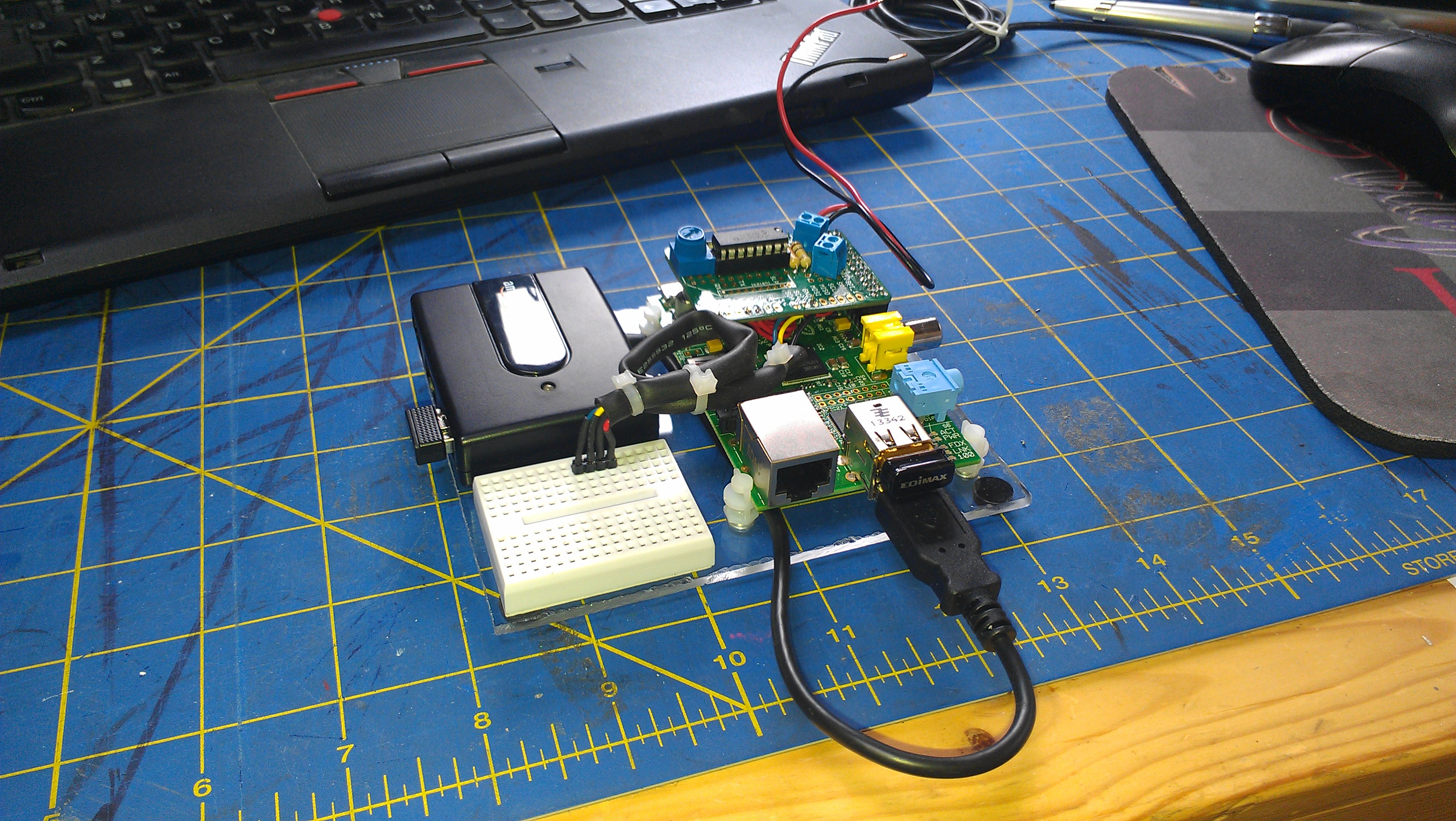All set, Raspberry PI B, USB Hub, and a breadboard.
The connectors plugged on the breadboard are I2C ones.
I used it with a BMP180 (Air temperature & Pressure).

Note: Since this project begun, the Raspberry PI B+ has been released. As it has 4 USB ports, you would not need the USB Hub featured above. This would allow a smaller setting, or a bigger breadboard.

## The budget

As of April 2014, here is what it takes:

 A Slice of PI \$ 9.05 from Amazon A 50K potentiometer \$ 0.95 from Adafruit An MCP3008 \$ 3.75 from Adafruit 2 connector terminals 5 for \$ 2.95 from Adafruit Resistors, wires, etc Total \$ 16.70

## Schema

Fritzing schema available here.

Oliv did it